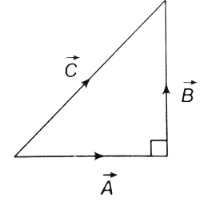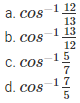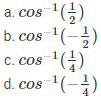# Addition and Subtraction of Vectors MCQs for NEET

NEET  Physics is the scoring paper in the medical entrance examination. Here, you will discover the NEET Physics MCQ Questions for all Concepts as per the latest syllabus. Practice more on a regular basis with these NEET Physics objective questions on air pollution and improve your subject knowledge & problem-solving skills along with time management. NEET Physics Addition and Subtraction of Vectors Multiple Choice Questions make you feel confident in answering the question in the exam & increases your scores to high.

## MCQ on Addition and Subtraction of Vectors

1. At what angle should the two force vectors 5N and 12 N be added to get a resultant vector of 13 N?
(a) 0°
(b) 30°
(c) 90°
(d) 180°

2. If the sum of two unit vectors is 1, then what is the difference between them?
(a) $$\frac { 1 }{ \sqrt { 2 } }$$
(b) √2
(c) √3
(d) √4

3. What is the net force on the particle if it is simultaneously acted upon by two forces 4 N and 3 N?
(a) 1 N
(b) 7 N
(c) 21 N
(d) Between 7 N and 1 N

Answer: (d) Between 7 N and 1 N

4. If the magnitude of the resultant force of two forces is lesser than the magnitude of the larger force, then
(a) The two forces are perpendicular to each other
(b) They two forces in opposite directions
(c) The forces are different in magnitude and direction
(d) The forces possess small magnitude

Answer: (b) The two forces are in opposite directions

5. Two forces $$\overrightarrow { { F }_{ 1 } }$$ and $$\overrightarrow { { F }_{ 2 } }$$ that are perpendicular to each other act on a point mass. The resultant force in the point mass is given by
(a) F1=F2
(b) F1−F2
(c) $${ F }_{ 1 }^{ 2 }+{ F }_{ 2 }^{ 2 }$$
(d) $$\sqrt { { F }_{ 1 }^{ 2 }+{ F }_{ 2 }^{ 2 } }$$

Answer: $$\sqrt { { F }_{ 1 }^{ 2 }+{ F }_{ 2 }^{ 2 } }$$

6. If $$\left| \overrightarrow { A } -\overrightarrow { B } \right| =\left| \overrightarrow { A } \right| =\left| \overrightarrow { B } \right|$$, then what is the angle between $$\overrightarrow { A }$$ and $$\overrightarrow { b }$$ ??
(a) 0°
(b) 30°
(c) 60°
(d) 90°

7. Which of the following is true for the given diagram?Answer: (b) $$\overrightarrow { C } +\overrightarrow { A } =\overrightarrow { B }$$

8. If three vectors A, B and C have magnitudes 5, 12 and 13 and $$\overrightarrow { A } +\overrightarrow { B } =\overrightarrow { C }$$, then what is the angle between B and C?Answer: (a) $$\cos ^{ -1 }{ \frac { 12 }{ 13 } }$$

9. Two forces F1 and F2 act on a body. One of the forces is double of the other force. The resultant of these forces is equal to the larger force. What is the angle between the forces?Answer: (d) $$\cos ^{ -1 }{ -\frac { 1 }{ 4 } }$$